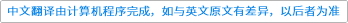Journal of Computational and Applied Mathematics ( IF 2.037 ) Pub Date : 2020-12-30 , DOI: 10.1016/j.cam.2020.113364

Implicit schemes for solving large-scale linear differential–algebraic systems with constant coefficients necessitate at each integration step the solution of a linear system, typically obtained by a Krylov subspace method such as GMRES. To accelerate the convergence, an approach is proposed that computes good initial guesses for each linear system to be solved in the implicit scheme. This approach requires, at each integration step, a small dimensional subspace where a good initial guess is found using the Petrov–Galerkin process. It is shown that the residual associated with the computed initial guess depends on the dimension of the subspace, the order of the implicit scheme, and the discretization stepsize. Several numerical illustrations are reported.down
wechat
bug